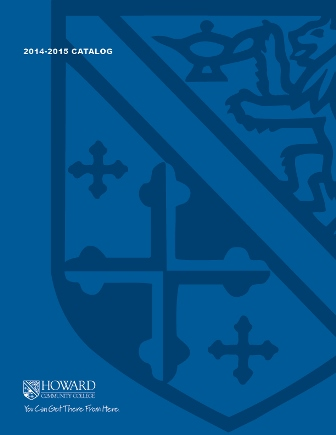2017-2018 College Catalog# MATH 153 Precalculus II

Students will develop skills in basic trigonometry and its applications, with an emphasis on modeling with functions and other algebraic skills necessary for the study of calculus. A graphical approach will be utilized throughout, with an emphasis on solving application problems. Trigonometry will be defined using the unit circle approach, with emphasis on classical right triangle trigonometry, forming the basis for learning how to use trigonometric identities and solve trigonometric equations, graph and use properties of the trigonometric functions and their inverses, parametric equations, use polar coordinates, and use complex numbers, including DeMoivre's theorem. Additional topics from algebra will include solving absolute value and quadratic inequalities, solving higher-order polynomials, and using sequences and series. The use of a graphing calculator is required.

3

### Prerequisite

MATH 143 with a grade of C or higher

3 hours weekly

### Course Objectives

1. 1. Communicate mathematical concepts related to pre-calculus topics using appropriate
symbols, notation, and vocabulary.
2. 2. Solve application problems by correctly applying appropriate pre-calculus methods,
theorems, and/or models (such as operations on, and transformations of, trigonometric
functions).
3. 3. Identify and implement appropriate technologies to efficiently complete tasks that involve the
solving of cross-discipline, mathematically appropriate problems and creating new works to
communicate the processes used and solution.
4. 4. Analyze, evaluate, justify, and interpret the reasonableness of solutions to precalculus
problems.

### Course Objectives

1. Communicate mathematical concepts related to pre-calculus topics using appropriate
symbols, notation, and vocabulary.

2. Solve application problems by correctly applying appropriate pre-calculus methods,
theorems, and/or models (such as operations on, and transformations of, trigonometric
functions).

3. Identify and implement appropriate technologies to efficiently complete tasks that involve the
solving of cross-discipline, mathematically appropriate problems and creating new works to
communicate the processes used and solution.

4. Analyze, evaluate, justify, and interpret the reasonableness of solutions to precalculus
problems.